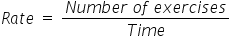General
General
Easy

Question

# Fanny can solve 5 math exercises in an hour. At the same rate, 25 math exercises take ______ hours.

## 6957Hint:

## The correct answer is: 5

### The given value of number of exercises is 5 and time required is 1 hour.Basically we are given a rate at which Fanny is solving the exercise. It is 5 exercises per hour.We have to find time taken by him to solve 25 exercises. We have rate with us and it is constant. So we will use to the time required.The equation becomesWe will rearrange the expression for time. We will divide the L.H.S and R.H.S of the above equation by rate and multiply it by time.Therefore, Fanny requires 5 hours to solve 25 math exercises.

The other method is to take the ratios and directly equate them. As the rate is constant, so the ratios will be constant. So we will consider the quantity we have to find as x and then equate the ratios. It will be a shortcut.

### Related Questions to study#### With Turito Foundation.#### Get an Expert Advice From Turito.